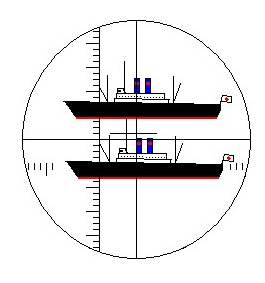Quote of the Day

In my many years I have come to a conclusion that one useless man is a shame, two is a law firm, and three or more is a congress.

## IntroductionFigure 1: Example of the View of a Target
Using a Submarine Periscope's

Recently, I was reading about stadiametric range finding methods being used by hunters and their telescopic sights – I was surprised to find a lot of writing on the topic. As I researched the topic, I saw that there are three common approaches used in telescopic sights: milliradian (mil), Minute Of Angle (MOA), and Inch Of Angle (IOA). I will review these methods here.

My interest in these methods comes from my addiction to a video game, Silent Hunter, which is an excellent simulation of submarine warfare during WW2. Periscopes contain a built-in stadimeter (Figure 1) that was used to measure the angular height of a target ship. If the target ship's height could be obtained from nautical reference manuals, the range to the target ship could be computed using the formula$R={}^{h}\!\!\diagup\!\!{}_{\theta }\;$.

## Background

The following Youtube video (Figure 2) does a nice job of reviewing the calculation mechanics of stadiametric range determination using a telescopic sight. However, it does not derive the formulas that are used. I will derive these formulas in the Analysis portion of this post.

As an engineer, I view all three methods as variations on the same theme. However, the topic is a subject for some debate because stadiametric ranging using these sights is one of the few areas where people still routinely do arithmetic in their head. If you are a person who likes to work with inches, the arithmetic may be simpler for the MOA and IOA methods because of some useful measurement coincidences. If you work in the metric system, you may find the milliradian approach has simpler math. It all depends on how your brain is wired.

## Analysis

The milliradian (mil) is an approach that can be used regardless of the unit of measure. It is based on the use of radian unit, which is the standard unit of angular measure in the mathematical world. Figure 3 shows how to derive the distance subtended by one mil at 100 yards and the range at which 1 inch is subtended by a mil.

On a personal note, the mil is actually the first unit of angular measure that I learned as boy. My dad was an old US Army artilleryman and he liked to use these units. Don't get me started on the differences between the different definitions of mil – so many definitions and so little difference.

We can determine the range of a 12-inch target with a circular measure of 1.6 mils as shown in Figure 4.

### True Minute of Angle

Figure 5 shows that a minute of angle subtends an arc of 1.0472 inches at 100 yards or 1.00 inches at 95.5 yards . Most people approximate both of these relationships as 1 inch at 100 yards. For accurate ranging at long distance, you need to account for the 4.7% of error.

To illustrate how to use a telescopic sight with minute-of-angle graduations, consider the case where we have a 12-inch objects that subtends 1.6 MOA in the sight. We can compute the range of this object as shown in Figure 6.

### Shooter's Minute of Angle (SMOA) or Inch of Angle (IOA)

In actual fact, you can define a circular angle in any number of ways. A small number of telescopic sights define the circular angle in terms of the angle subtended by 1 inch at 100 yards (Figure 7). This makes the math very simple for those who like to think in terms of inches.

I will again work an example (Figure 8) that assumes a 12-inch target but that now subtends 1.6 IOA.

## Conclusion

I was amazed at the number of forum posts on this topic that I encountered. At a fundamental level, these approaches are all mathematically equivalent. It really comes down to what is more comfortable for the individual. If your mind is really into customary units like inches, the IOA/SMOA seems like the best. The mil-radian approach is unit-independent and that has its attractions. I am unclear as to why anyone would want to use the true MOA approach, however, some reticles are not available in the IOA format and you may have no choice.

This entry was posted in Ballistics, General Mathematics. Bookmark the permalink.

### 2 Responses to Stadiametric Rangefinding Approaches

1.Randy says:

Mark,

The vast majority of reticles are not available in IOA format. History is the major determinant of this outcome. The technology was passed from the military to the sportsman, and that's what stuck.

2.Osmond Lin says:

I am looking for a simple software outsourcing for stadiametric range finder for our monocular. If you are interested for extra income, please contact me as soon as possible. Thanks!